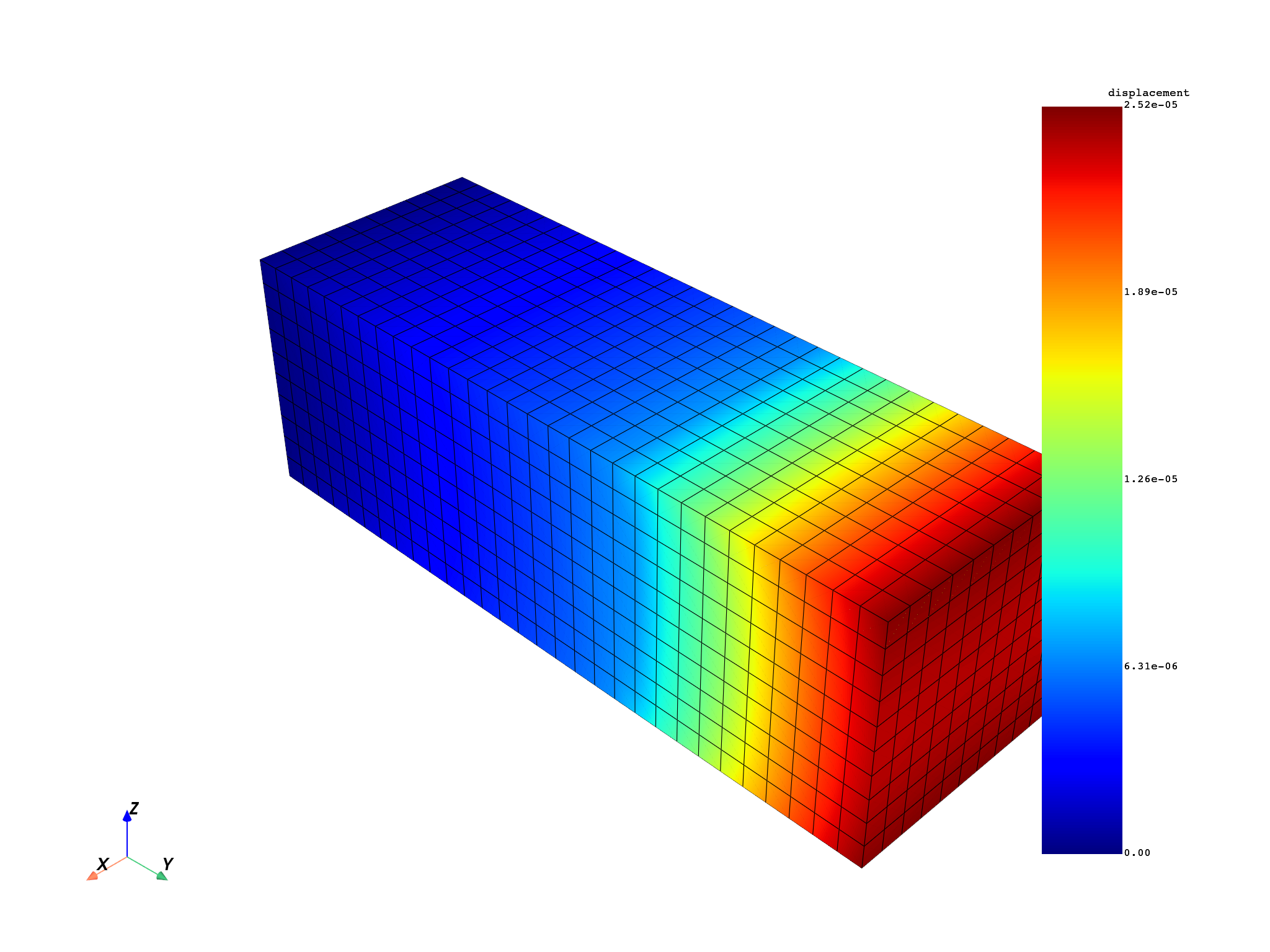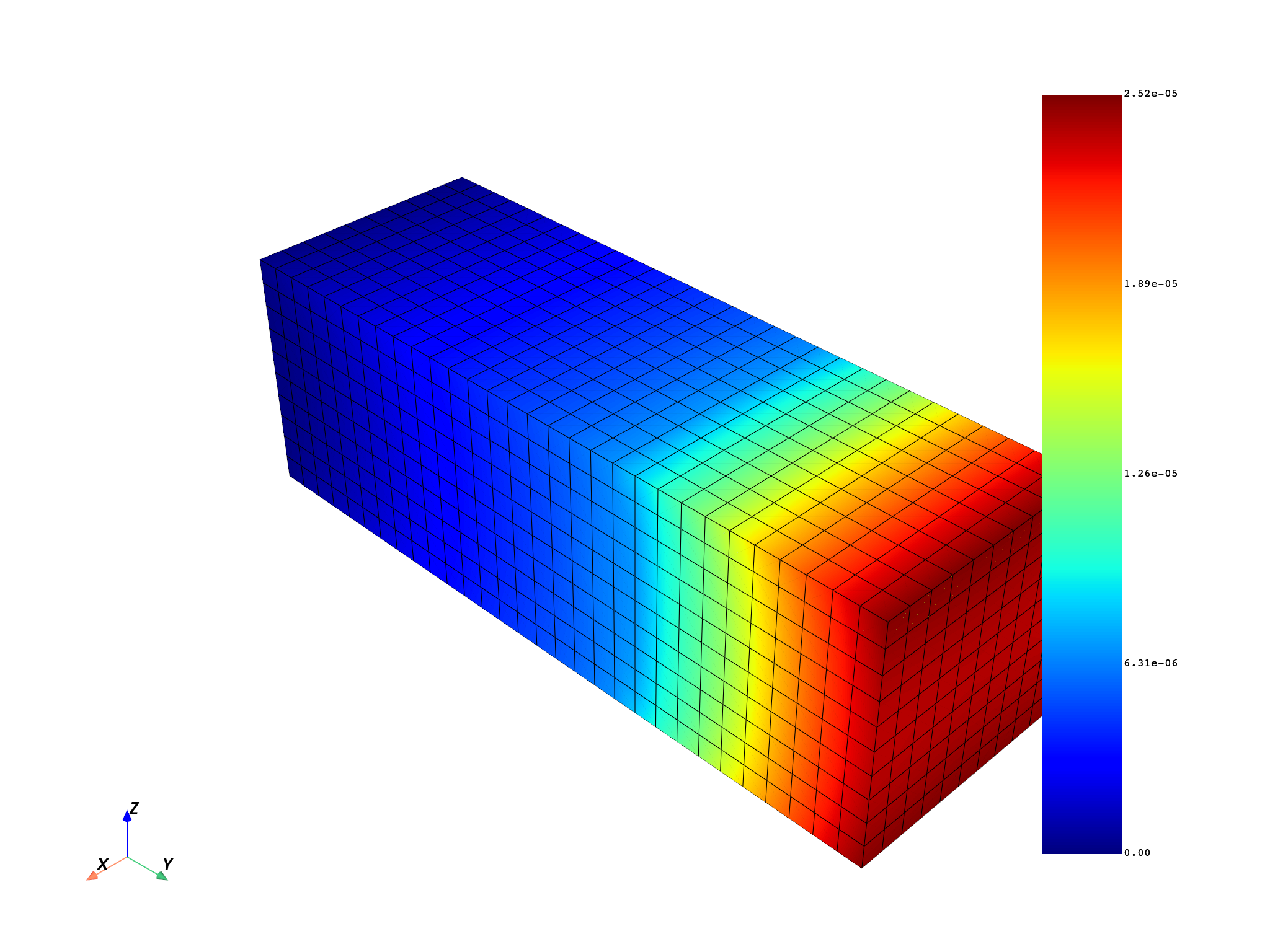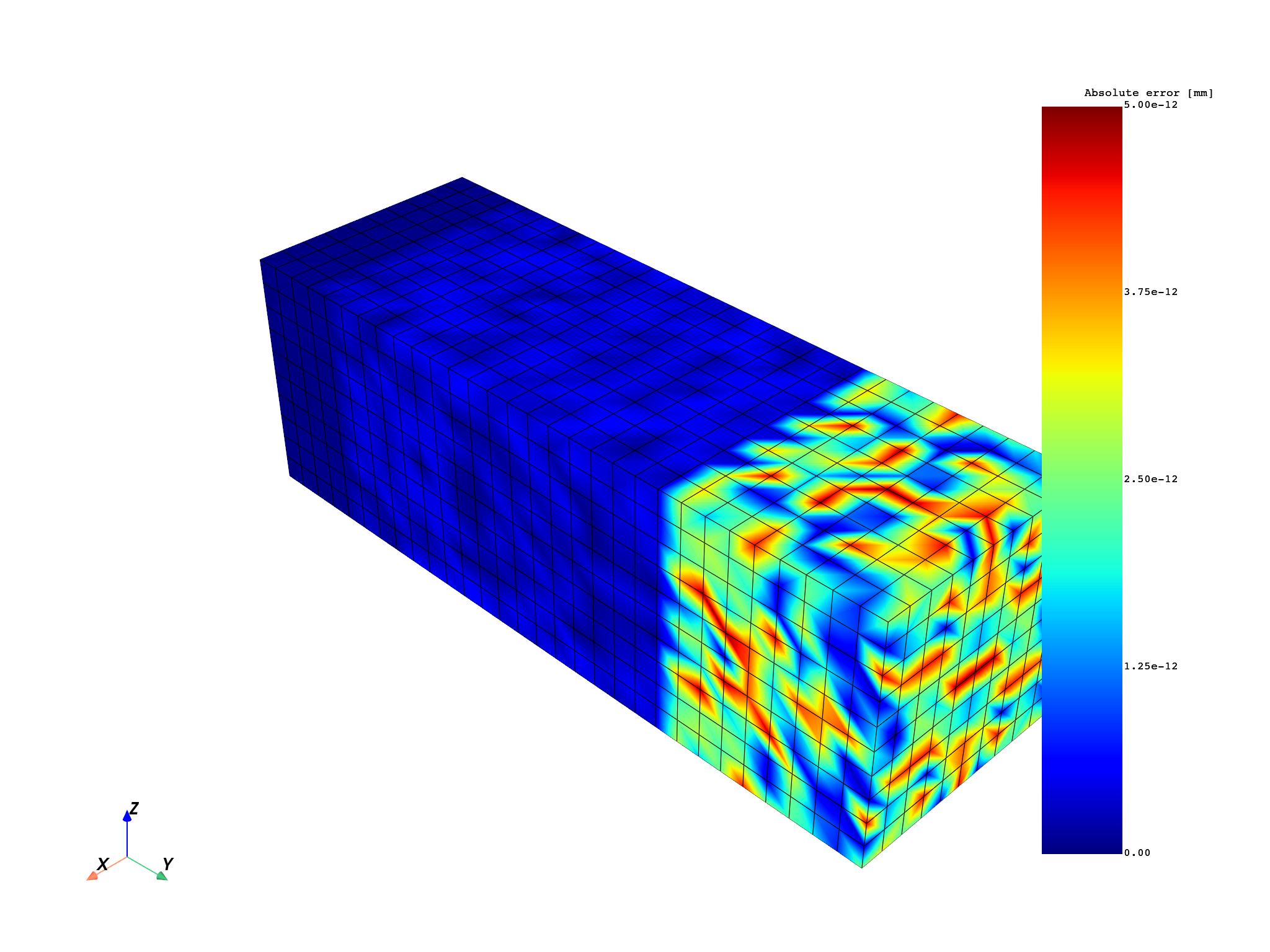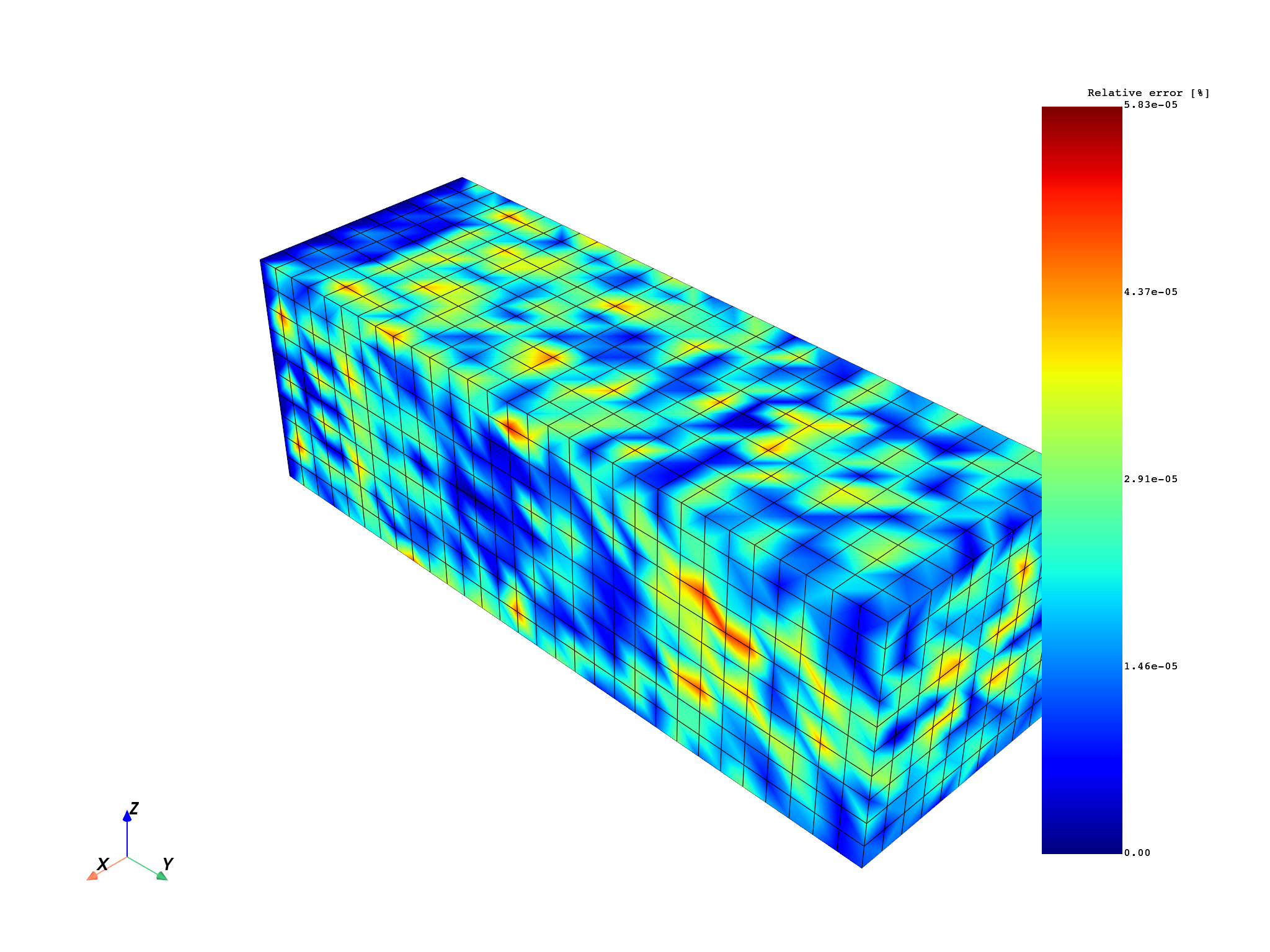# Working with a result file#

This example shows how to write and upload files on the server machine and then download them back on the client side. The resulting fields container is then exported to a CSV file.

Note

Load a model from the DPF-Core examples: `ansys.dpf.core` module.

```from ansys.dpf import core as dpf
from ansys.dpf.core import examples

model = dpf.Model(examples.find_simple_bar())
```

# Get and plot the fields container for the result#

Get the fields container for the result and plot it so you can compare it later:

```displacement_operator = model.results.displacement()
fc_out = displacement_operator.outputs.fields_container()
mesh.plot(fc_out)
```# Export result#

Get the fields container for the result and export it in the CSV format:

```import os

file_path = os.getcwd() + "\\simple_bar_fc.csv"

export_csv_operator = dpf.operators.serialization.field_to_csv()
export_csv_operator.inputs.field_or_fields_container.connect(fc_out)
export_csv_operator.inputs.file_path.connect(file_path)
export_csv_operator.run()
```

Upload the file `simple_bar_fc.csv` on the server side. Here, `upload_file_in_tmp_folder()` is used because it is assumed that the server machine architecture is unknown. However, when the server file path is known, `upload_file()` can be used.

```if not dpf.SERVER.local_server:
print(server_file_path)

# Remove file to avoid polluting.
os.remove(file_path)
```

Download the file `simple_bar_fc.csv`:

```if not dpf.SERVER.local_server:
else:
```

# Load CSV result file as operator input#

Load the fields container contained in the CSV file as an operator input:

```my_data_sources = dpf.DataSources(downloaded_client_file_path)
import_csv_operator = dpf.operators.serialization.csv_to_field()
import_csv_operator.inputs.data_sources.connect(my_data_sources)

# Remove file to avoid polluting.
```# Make operations over the imported fields container#

Use this fields container to get the minimum displacement:

```min_max_op = dpf.operators.min_max.min_max_fc()
min_field = min_max_op.outputs.field_min()
min_field.data
```
```DPFArray([[-8.202171e-07, -6.265107e-06, -2.444680e-05]])
```

Subtract the two fields and plot an error map:

```abs_error = (fc_out - downloaded_fc_out).eval()

divide = dpf.operators.math.component_wise_divide()
divide.inputs.fieldB.connect(fc_out)
scale = dpf.operators.math.scale()
scale.inputs.field.connect(divide)
scale.inputs.ponderation.connect(100.0)
rel_error = scale.eval()
```

# Plot both absolute and relative error fields#

Note that the absolute error is bigger where the displacements are bigger, at the tip of the geometry. Instead, the relative error is similar across the geometry since we are dividing by the displacements `fc_out`. Both plots show errors that can be understood as zero due to machine precision (1e-12 mm for the absolute error and 1e-5% for the relative error).

```mesh.plot(abs_error, scalar_bar_args={"title": "Absolute error [mm]"})
mesh.plot(rel_error, scalar_bar_args={"title": "Relative error [%]"})
```
••Total running time of the script: ( 0 minutes 5.540 seconds)

Gallery generated by Sphinx-Gallery